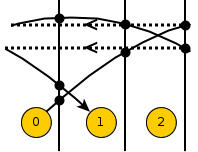# ICL1703 - Editorial

Practice

Contest

Author: Eklavya Sharma

Tester: Ankit Sultana

Editorialist: Eklavya Shama

Medium

# Prerequisites

Modular arithmetic

# Explanation

We have to color a graph’s edges under the following constraints:

• colors of all outgoing edges are distinct.
• colors of all incoming edges are distinct.
• set of colors of incoming edges is the same as the set of colors of outgoing edges.

The problem has 2 parts:

1. checking if the constraints can be satisfied.
2. finding out the maximum number of colors if the constraints can be satisfied.

### Part 1 - Checking if constraints can be satisfied

For a graph satisfying the above constraints, the graph can be represented as union of
multiple infinitely-long chains.

Constraints will be satisfied iff for every vertex indegree is equal to outdegree.
Outdegree of a vertex is the size of its label array.
So we can find the indegree of every vertex and compare with the outdegree.

Consider a vertex v. Let its indegree be d.

That means that there exist pairs of integers (u1, w1),
(u2, w2), …, (ud, wd) such that
wi is a number in the label of vertex ui and
ui + wi = v for all i from 1 to d.

On taking mod n, we find that ui + wi ≡ v (mod n) for all i from 1 to d.

Similarly, if there exist exactly d pairs of integers (u1, w1),
(u2, w2), …, (ud, wd) such that
wi is a number in the label of vertex ui and
ui + wi ≡ v (mod n) for all i from 1 to d,
then indegree of vertex v is d.
(Because ui + wi ≡ v (mod n) implies (ui+ k * n) + wi = v)

Indegrees are also periodic with period n. We’ll find the indegree of vertices 0 to n-1.

Make an array D of size n. D[i] will finally hold the indegree of vertex i.
D[i] is initially 0 for all i.

``````for all u from 0 to n-1:
for all w in label of vertex u:
D[(u + w) % n] += 1
``````

This will give us indegree of vertex i in D[i].

Compare D[i] with size of label of vertex i.
If they are the same for all i, then the graph satisfies constraints.

### Part 2 - Finding out maximum number of colors

The maximum number of colors is the number of infinite chains in the graph.

Make a vertical line between every vertex i and vertex i+1. Let’s call this the ith ‘border’.
Also partition the graph into partitions of size n, where the ith partition has the borders
numbered n*i to n*i + (n-1) and vertices numbered n*i to n*i + (n-1).

The number of chains is equal to the number of edges which a border intersects.
We can see that the number of intersections will be the same for every border
if indegree and outdegree of every vertex is the same (which is given in constraints).

To calculate the number of chains, we’ll sum the number of intersections at borders 0 to n-1 and divide by n.
(Since they’re equal, it might seem counter-intuitive to take average, but you’ll see later why I did it this way).

An edge from u (0 ≤ u < n) to u+w will intersect w borders: u, u+1, …, u+w-1.
Some of these borders lie in partition 0 while some may lie in higher partitions.
So, a border might be intersected by edges from it’s own partition and edges from previous partitions.

Assume that a border numbered t (0 ≤ t < n) is intersected by edges from vertex u, u-n, u-2n, …, u-kn (0 ≤ u < n).
We can have the same effect if we only consider a graph with n vertices and wrap all edges.

#### What it means to wrap a graph

Consider a graph with periodicity 3.
For the first vertex of each partition, let 7 be an element in its label.
Consider a subgraph which only has these edges (i.e. an edge from 3n to 3n+7 for all n).

This will be its wrapped graph:In the wrapped graph, all borders will have the same number of intersections as in the original graph.
The number of intersections made by an edge is equal to the length of the edge in the wrapped graph too.

Therefore, the total number of intersections made by edges originating from partition 0 is equal to
the sum of lengths of all edges. This is the same as the sum of all elements in all arrays.

Therefore, the number of intersections at each border is equal to the sum of all elements of all arrays
divided by n. This is the maximum number of colors needed to color the graph.

# Time complexity

Linear in the size of input.

# Solution codes:

Setter’s solution

Tester’s solution

1 Like

Can anyone proove why is sum of length of same type of edges in wraped graph equal to its orignal length?

//